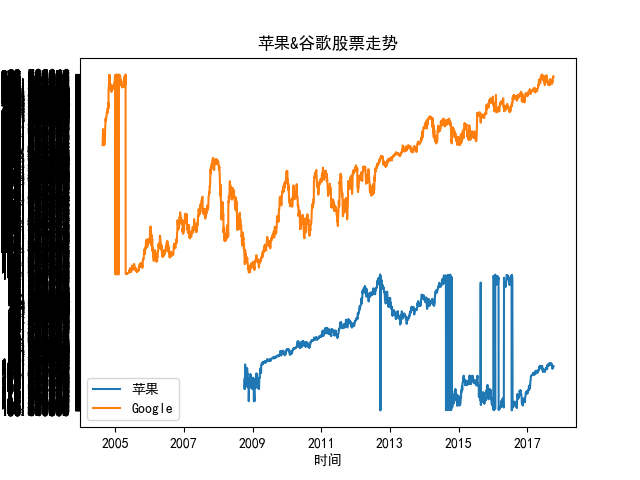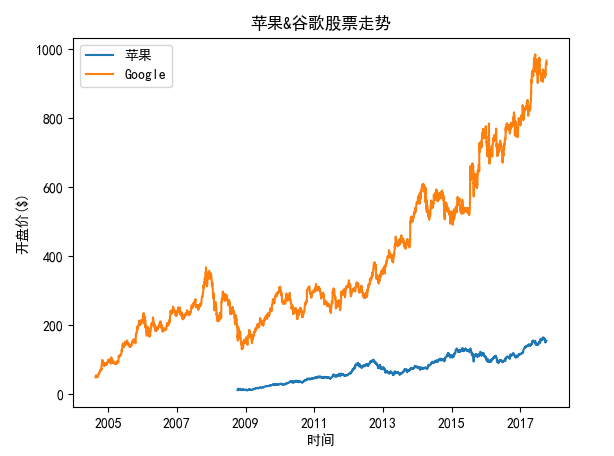# When use a large data to draw a graph, It shows abnormal.. #9360

Closed
opened this issue Oct 11, 2017 · 8 comments

Projects
None yet

### Bug report

Bug summary
When use a large data to draw a graph, It shows abnormal..The data is about 2300 rows.When use in a small scale data, it shows normally.

Code for reproduction

```  1 #!/usr/bin/env python3
2 # -*- coding:utf-8 -*-
3
4 import matplotlib.pyplot as plt
5 import matplotlib
6 import csv
7 import numpy as np
8 import matplotlib.dates as mdates
9 from datetime import datetime
10
11 def graph_data():
12     fig = plt.figure()
13     ax1 = plt.subplot2grid((1,1), (0,0))
14     stock_data = []
15     x1 = []
16     y1 = []
17     x2 = []
18     y2 = []
19     matplotlib.rcParams['font.sans-serif'] = ['simhei']
20     matplotlib.rcParams['axes.unicode_minus'] = False
21     with open('AAPL.csv', 'r') as csvfile:
24             stock_data.append(row)
25     del stock_data
26     for row in stock_data:
27         x1.append(row)
28         y1.append(row)
29     x1 = [datetime.strptime(d, '%Y-%m-%d').date() for d in x1]
30     stock_data = []
31     with open('GOOG.csv', 'r') as csvfile:
34             stock_data.append(row)
35     del stock_data
36     for row in stock_data:
37         x2.append(row)
38         y2.append(row)
39     x2 = [datetime.strptime(d, '%Y-%m-%d').date() for d in x2]
40     plt.plot(x1,y1,label='苹果')
42     plt.title('苹果&谷歌股票走势')
43     plt.xlabel('时间')
44     plt.ylabel('开盘价(\$)')
45     plt.legend()
46     plt.show()
47 graph_data()```

Actual outcome
This is the picture which output:Expected outcome
And this is the normal picture(with matplotlib 2.0.2):Matplotlib version

• Operating system:Ubuntu 16.04 LTS with Python3.5
• Matplotlib version: 2.1.0
• Matplotlib backend (`print(matplotlib.get_backend())`):
• Python version:
• Jupyter version (if applicable):TkAgg
• Other libraries: None

Member

### tacaswell commented Oct 11, 2017

 Why is the data different between the two graphs? Can you reproduce this with random data?
Contributor

### anntzer commented Oct 11, 2017

 Cannot reproduce with master or 2.0.2, after patching the code to `````` stock_data = np.load("aapl.npz") x1 = stock_data["price_data"]["date"] x1 = [x.astype(datetime) for x in x1] y1 = stock_data["price_data"]["open"] stock_data = np.load("goog.npz") x2 = stock_data["price_data"]["date"] x2 = [x.astype(datetime) for x in x2] y2 = stock_data["price_data"]["open"] `````` which should be exactly the same (run from the sample_data folder). OTOH I don't have simhei installed. Can you also reproduce with another font?
Author

### etveritas commented Oct 11, 2017

 I use two versions of matplotlib,the normal graph is about version 2.0.2,and the abnormal graph is about version 2.1.0.I draw with the smae data. there is anonther strange thing, when I use a small scale data with version 2.1.0, it can draw normally with the same code...@tacaswell
Author

### etveritas commented Oct 11, 2017

 You can delete the statement about the font setting,then change the label's language. @anntzer
Member

### tacaswell commented Oct 11, 2017

 Your y vectors are lists of strings, cast them to float before plotting and it should work. They are now being plotted as categorical values (see http://matplotlib.org/users/whats_new.html#string-categorical-values). As part of that we removed some (we thought) accidental behavior where lists of floats encoded as strings magically worked. You can also use `pandas` to load csv files which does a better job of guessing the correct types.
Author

### etveritas commented Oct 11, 2017

 ``` 1 #!/usr/bin/env python3 2 # -*- coding:utf-8 -*- 3 4 import matplotlib.pyplot as plt 5 import matplotlib 6 import csv 7 import numpy as np 8 import matplotlib.dates as mdates 9 from datetime import datetime 10 11 def graph_data(): 12 fig = plt.figure() 13 ax1 = plt.subplot2grid((1,1), (0,0)) 14 stock_data = [] 15 x1 = [] 16 y1 = [] 17 x2 = [] 18 y2 = [] 19 with open('AAPL.csv', 'r') as csvfile: 20 reader = csv.reader(csvfile) 21 for row in reader: 22 stock_data.append(row) 23 del stock_data 24 for row in stock_data: 25 x1.append(row) 26 y1.append(row) 27 x1 = [datetime.strptime(d, '%Y-%m-%d').date() for d in x1] 28 stock_data = [] 29 with open('GOOG.csv', 'r') as csvfile: 30 reader = csv.reader(csvfile) 31 for row in reader: 32 stock_data.append(row) 33 del stock_data 34 for row in stock_data: 35 x2.append(row) 36 y2.append(row) 37 x2 = [datetime.strptime(d, '%Y-%m-%d').date() for d in x2] 38 plt.plot(x1,y1,label='Apple') 39 plt.plot(x2,y2, label='Google') 40 plt.title('A&G') 41 plt.xlabel('Time') 42 plt.ylabel('Price(\$)') 43 plt.legend() 44 plt.show() 45 graph_data()``` About this.@anntzer
Author

### etveritas commented Oct 11, 2017

 OH,Thank you very much. I change ```y1.append(row) y2.append(row)``` to ```y1.append(float(row)) y2.append(float(row))``` It draws normal graph. Thanks! @tacaswell
Member

### tacaswell commented Oct 11, 2017

 Closing as 'wont fix' as this is hitting a new feature because the OP was using a code path we did not think was being used and if we had tried to maintain would have blocked implementation of string categorical s.